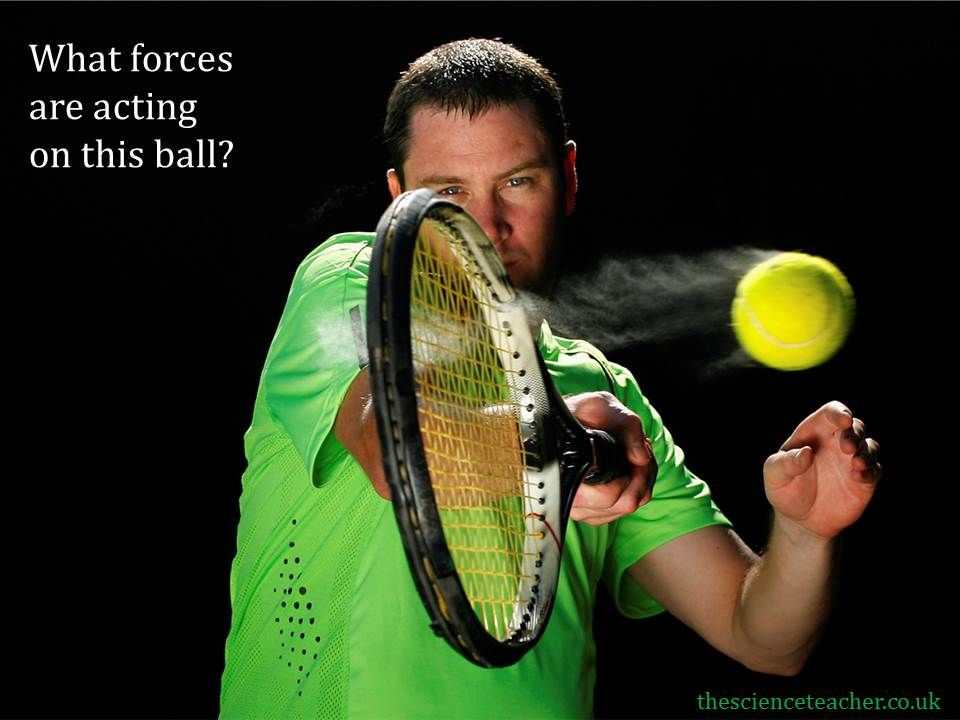# Newton’s laws teaching resources

###### Worksheets and lesson ideas to challenge students aged 11 to 16 to think hard about Newton’s laws (GCSE and Key Stage 3)

It’s really important that teachers spend time helping students to understand Newton’s Laws of Motion – and can use his framework to help scaffold thinking and explanations. Read Rosalind Driver for information on key misconceptions that must be planned for and remember that many of these student misconceptions will also be ingrained in us!

#### Thought experiments to see if students understand Newton’s laws of motion

GCSE and Key Stage 3 worksheet on Newton’s laws. Students can state Newton’s laws but do they understand them? Here we use a series of thought experiments to see if students understand Newton’s three laws of motion. This can also be used to support new teachers think about student misconceptions. (PDF)

####Newton’s first law of motion

Key Stage 3 and GCSE worksheet on Newton’s first law of motion. Students identify and label forces acting on a tennis ball that has been hit by a racket. Many students will mistakenly think there is a force acting in the direction of motion i.e. pushing the ball along. Of course there is not, only air resistance and weight are acting. (PDF)

#### Understanding Newton’s third law

GCSE and Key stage 3 worksheet on Newton’s third law. The purpose of this task is to help students understand Newton’s third law. It is quite common for students to think that if interacting forces act in pairs, then a force in one direction will be cancelled out by an equal force acting in the opposite direction. Of course, the interacting forces act on different objects and so both objects will experience the force. (PDF)